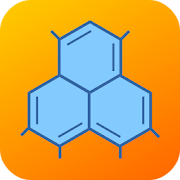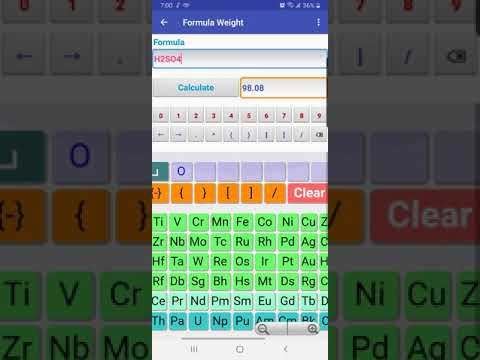# Stoichiometry PlusEveryoneThe Stoichiometry calculator provides three ways for reaction stoichiometric calculations:

Quick Stoichiometry, Reactant Amount Given, and Product Amount Given

Quick Stoichiometry calculates the stoichiometric amounts of all other substances when the amount of one substance, reactant or product, is given. Reactant Amount Given solves cases when one or more reactant amounts are given. Product Amount Given finds the amounts of all other substances when one product amount is given. Quick Stoichiometry uses units of mole or gram while Reactant Amount Given and Product Amount Given also accept inputs for gases, solutions, and liquids in addition to mole and gram.

For gas inputs, temperature can be Kelvin (K), Celsius (C) or Fahrenheit (F) and pressure units can be atm, kPa or psi. For inputs of solutions or liquids, there are choices of M, mM and g/ml.

Stoichiometric calculations require equation balance. The calculator will automatically balance equations for you if they are not balanced. If you already balance equations, the calculator will use your balance.

The equation balance calculator can balance equations for you. You can also use it to check results of your balancing.

The mole calculator or moler not only gives precise results but also shows calculation steps that lead to the results.

The solution preparation calculator helps you to prepare solutions from scratch or dilute existing solutions or liquids.

A convenient periodic table keyboard is provided. The keyboard remembers the most recently used elements so that you do not have to search for them again from the keyboard.

In addition to integers, both fractions and decimals are also accepted in formula.
Collapse

Review Policy

## What's New

A Solution Preparation calculator is added to the Stoichiometry Plus calculator collection
Collapse

Eligible for Family LibraryEligible if bought after 7/2/2016. Learn More
Updated
June 6, 2020
Size
2.9M
Installs
100+
Current Version
3.0
Requires Android
4.4 and up
Content Rating
Everyone
Permissions
Offered By
thermobook.net
Developer